# What is Algorithm

> What is Algorithm
Posted by Allison Lynch |
##### Want to Make Algrithm Flowcharts? Try This Out!

EdrawMax Flowchart Maker possesses with a huge amount of flowchart templates, you can edit and design a customized flowchart in a swift. Just try it for free now!

Switch to Mac >
Switch to Linux >
Switch to Windows >

In this passage, you will learn the basic knowledge of algorithm and know the types and common use of algorithm. Last but not the least, you would get to know the way to create Algorithm flowchart with ease! Keep reading!

## Part 1: What is an Algorithm?

In basic terms, an algorithm is a set of well-defined steps or rules that you need to follow to obtain a pre-determined result. For instance, when we talk about algorithms in computer programming, we already have our input and we know the expected output. Now, an algorithm would be all the defined steps to follow on the input to get the desired output.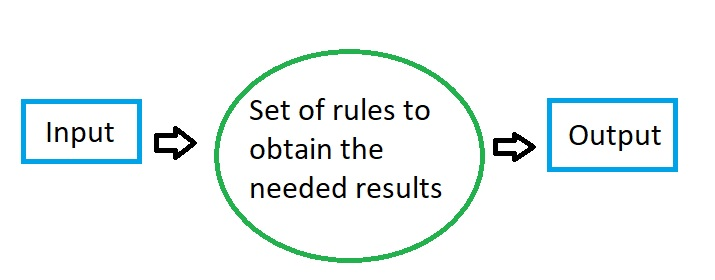Therefore, the entire algorithm flowchart would have three major components:

1. Input: What we already know or the things we have to begin with.
2. Algorithm: A set of sequenced steps that we need to follow one by one.
3. Output: The expected results we need to achieve in the end.

Apart from mathematics or computer programming, we see algorithms in everyday life. Let’s say you want to cook a dish. Now, you can begin with some ingredients (the input) and will follow the recipe. The recipe will have different steps (the algorithm) that you can follow to make the dish (the output).

## Part 2: Different Types of Algorithms

Before we learn how to create an algorithm flowchart, it is important to know that they can be classified in different forms. Therefore, you can explore the following types of algorithms and learn how to implement them in possible scenarios.

Brute force algorithm: This is the most common type in which we devise a solution by exploring all the possible scenarios.

Greedy algorithm: In this, we make a decision by considering the local (immediate) best option and assume it as a global optimal.

Divide and conquer algorithm: This type of algorithm will divide the main problem into sub-problems and then would solve them individually.

Backtracking algorithm: This is a modified form of Brute Force in which we backtrack to the previous decision to obtain the desired goal.

Randomized algorithm: As the name suggests, in this algorithm, we make random choices or select randomly generated numbers.

Dynamic programming algorithm: This is an advanced algorithm in which we remember the choices we made in the past and apply them in future scenarios.

Recursive algorithm: This follows a loop, in which we follow a pattern of the possible cases to obtain a solution.

##### Here to Create Algorithm Charts in Few Steps! Try Now!

EdrawMax Flowchart Maker visualizes the flow of every decision and system with a large variety of flowchart templates. Not only to drase the algorithm charts, you can also use other pre-made templates to design multiple flowcharts! Free to try!

Switch to Mac >
Switch to Linux >
Switch to Windows >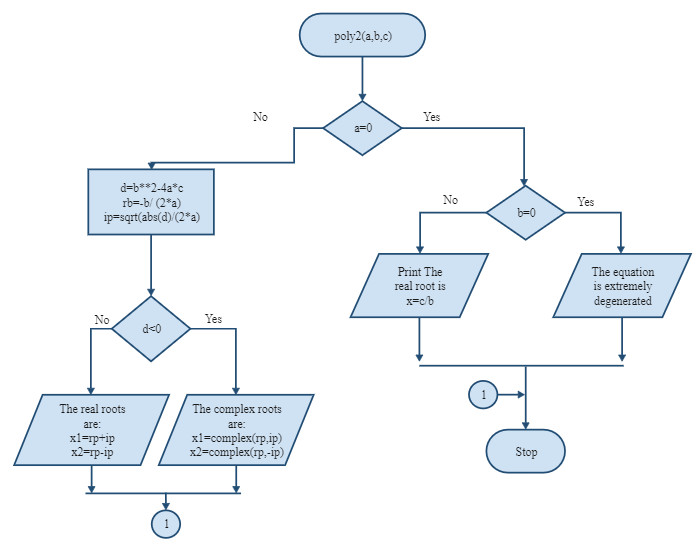## Part 3: The Common Applications of Algorithms

As listed above, algorithms are extremely important and can be applied in different fields. From computer programming to scientific experiments, algorithms have found a vital place in several domains. Ideally, you can apply an algorithm flowchart in the following ways.

In Computer Programming

It doesn’t matter which programming language you are using, the first thing you need to solve a problem is by coming up with an algorithm. Even the most complex of problems can be resolved by working on an algorithm flowchart.

Let’s consider that we have to write a program to automatically print numbers from 1 to 20. To do this, we initialize a variable and would perform an increment of 1 in every cycle. The cycle would be repeated till the number reaches 20.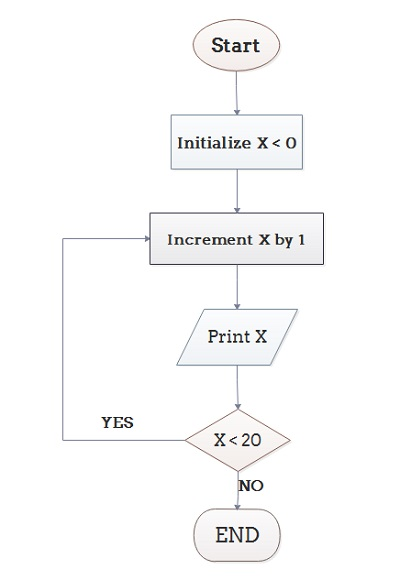Draw a flowchart for computing factorial N (N!).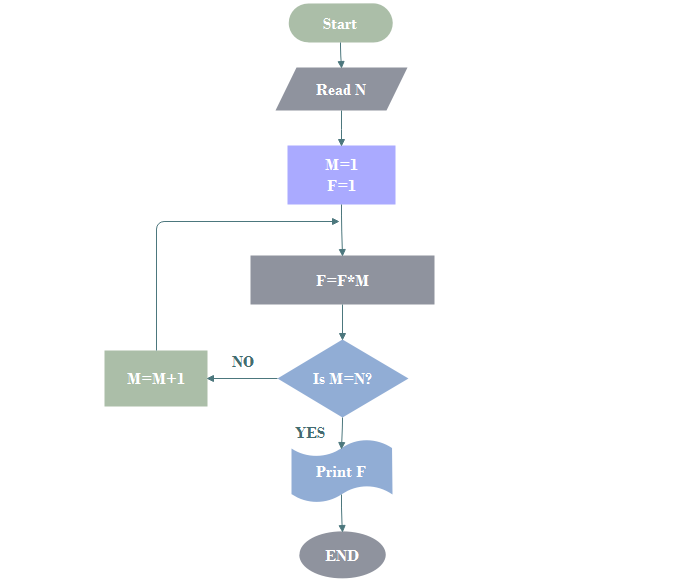In Mathematics and Scientific Calculations

Just like programming, algorithms can also be used in solving mathematical or scientific problems. From printing the Fibonacci series to calculating the factorial of a number, you can come up with an algorithm for any math problem.

In this example, you can see we have come up with an algorithm to convert Fahrenheit to Celsius. At first, we have taken the input from the user, applied the Fahrenheit to Celsius formula, and then would display the results.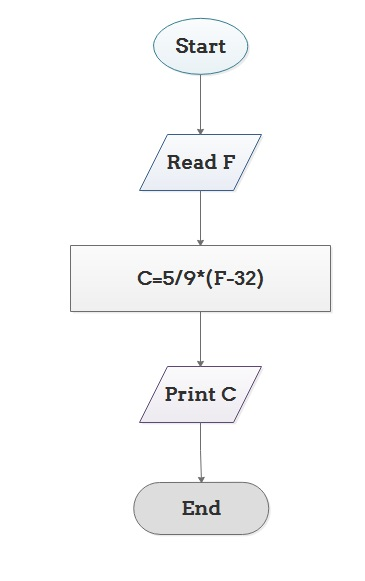Determine and Output Whether Number N is Even or Odd.Solving Everyday Problems

Besides programming or scientific problems, algorithms can be used to resolve almost every issue. You can use an algorithm to come up with a recipe, calculate tax, or any other problem.

Let’s say you want to calculate the grades of students and decide if they fail or pass. In this case, a flowchart like this can be followed to help you grade your students.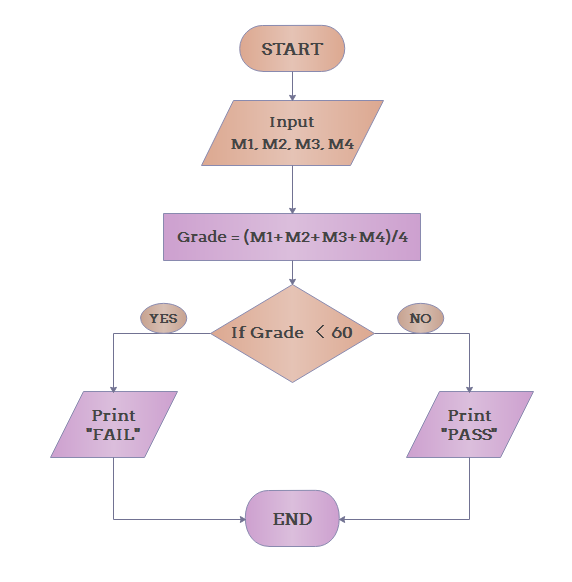## Part 4: How to Create an Algorithm Flowchart like a Pro?

Great! Now when you are familiar with the basics of algorithms, let’s learn how to create them. Ideally, I would recommend using a professional tool like Wondershare EdrawMax that can help you make all sorts of diagrams in a jiffy. The application has tons of diagramming tools and vectors to explore and even features an extensive set of templates that you can readily use.

#### EdrawMax

##### Efficient Algorithm Flowchart Maker >>
Provide professional flowchart templates for beginners and highly-customized drawings experience for experts
Create more than 280 types of diagrams effortlessly
• Superior file compatibility: Import and export drawings to various file formats, such as Visio
• Cross-platform supported (Windows, Mac, Linux, Web)Security Verified | Switch to Mac >>

To create different styles of algorithm flowcharts with the help of Wondershare EdrawMax, you can follow these steps:

Step 1: Select the Flowchart Module

To start with, you can just launch Wondershare EdrawMax and work on a new project. From the type of diagrams, select Flowchart > Basic Flowchart or any other diagram type. You can also load an existing template from the EdrawMax library to make your job easier.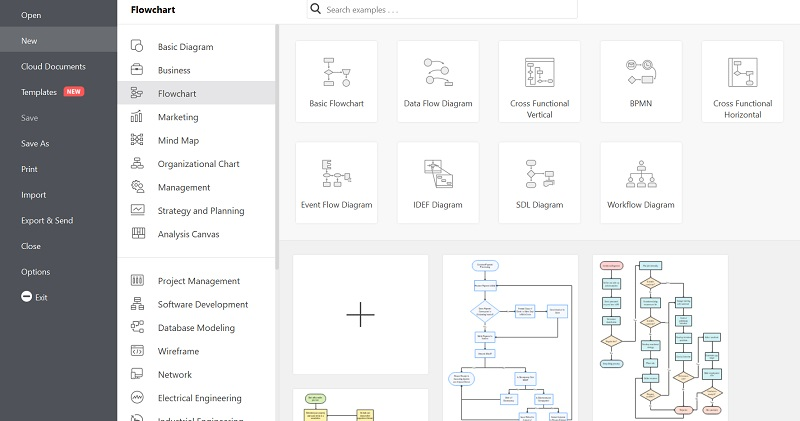Step 2: Create an Algorithm Flowchart

Let’s consider that we are making a flowchart to decide whether the temperature is below the freezing point or not. On the sidebar, you can see various vectors that are used to make an algorithm flowchart. You can drag-and-drop the icons to start the flowchart and input the temperature.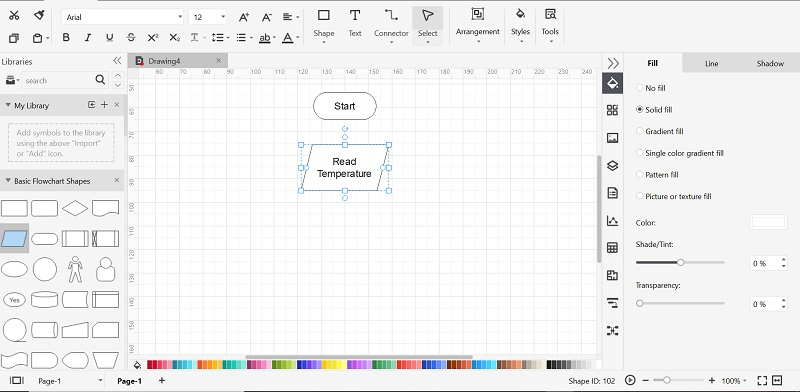Once you have read the temperature, you can check if it is below 32-degrees Fahrenheit or not. In this way, you can tell if the temperature is below or above the freezing point and inform the same to the user.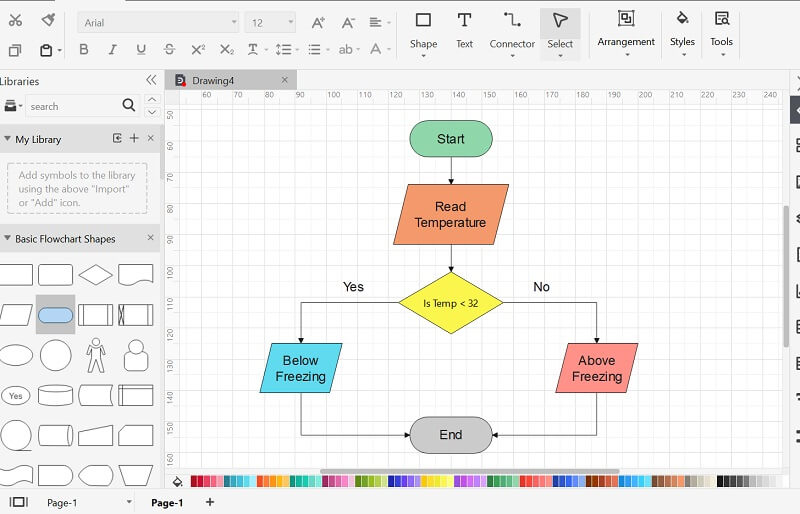Step 3: Export the Algorithm Flowchart

There are several other features in EdrawMax that would let you customize the flowchart and change its appearance. In the end, you can go to its Menu > Export to save your algorithm flowchart in the format of your choice.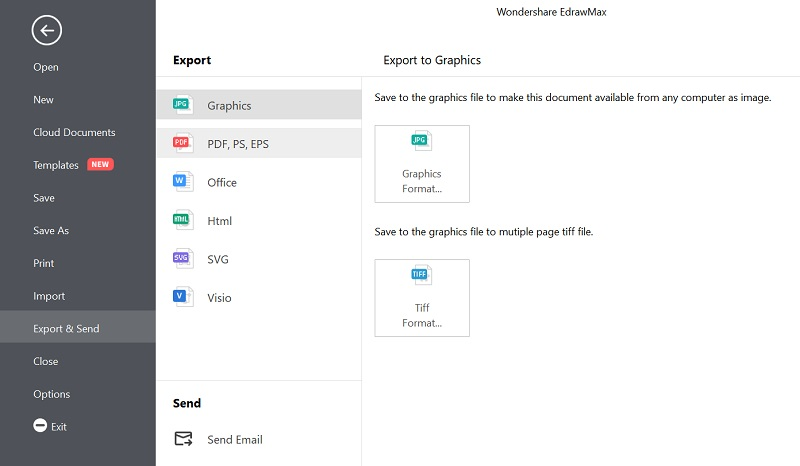There you go! Now when you know what an algorithm is and its types, you can easily solve any problem. In this post, I have also included the common applications and usage of algorithms to help you. Though, if you want to make intuitive and informative algorithm flowcharts, then simply use Wondershare EdrawMax. A resourceful diagramming tool, it will help you come up with all kinds of flowcharts and algorithmic diagrams in less time.

#### You May Also Like## Get Started! You Will Love This Easy-To-Use Diagram Software

EdrawMax is an advanced all-in-one diagramming tool for creating professional flowcharts, org charts, mind maps, network diagrams, UML diagrams, floor plans, electrical diagrams, science illustrations, and more. Just try it, you will love it!